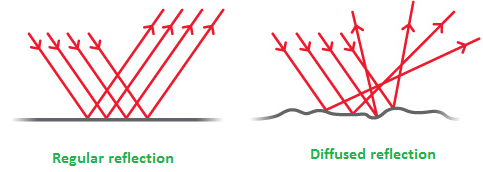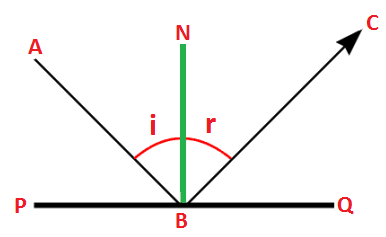# Introduction to Reflection of Light

Optics: The branch of physics which deals with the study of light is called optics.

Reflection of light: The phenomenon of bending of light without the change of its medium is called reflection of light. There are two types of reflection in general. When light strikes on the plane surface, it experiences regular reflection but when it strikes on the rough surface, light is reflected randomly. This reflection is called diffused reflection.Regular and diffused reflection

Some terms related with the reflection of lightIncident ray: It is the ray approaching to any surface. In figure above, AB is the incident ray.

Reflected ray: It is the ray which is reflected back after bouncing the surface. In figure, BC is the reflected ray.

Normal: The perpendicular drawn on the reflecting surface is called normal. In figure BN is the normal.

Reflecting surface: The surface which sends back the incident light is known as reflecting surface. In figure, PQ is the reflecting surface.

Angle of incidence: The angle made by the incident ray with the normal is the angle of incidence. In figure, ∠ABN is the angle of incidence.

Angle of reflection: The angle made by the reflected ray with the normal is called the angle of reflection. In figure, ∠NBC is the angle of reflection.

Note: Mirror has almost 100% reflecting capacity.
Laws of reflection:
• The incident ray, the reflected ray and the normal lies in the same plane.
• Angle of incidence is equal to the angle of reflection. In figure above, ∠ABN = ∠NBC are equal.

Do you like this article ? If yes then like otherwise dislike : 1

#### One Response to “Introduction to Reflection of Light”

1.Sunil

Laws of reflection